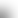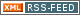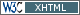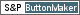www.LinuxHowtos.org howtos, tips&tricks and tutorials for linuxfrom small one page howto to huge articles all in one place

search text in:

Poll
Which kernel version do you use?

poll results

using iotop to find disk usage hogs

using iotop to find disk usage hogs

words:

887

views:

169916

userrating:

average rating: 1.7 (102 votes) (1=very good 6=terrible)

May 25th. 2007:
Words

486

Views

249274

Workaround and fixes for the current Core Dump Handling vulnerability affected kernels

Workaround and fixes for the current Core Dump Handling vulnerability affected kernels

words:

161

views:

135602

userrating:

average rating: 1.4 (41 votes) (1=very good 6=terrible)

April, 26th. 2006:

You are here: manpages

# float.h

Section: POSIX Programmer's Manual (0P)
Updated: 2013

## PROLOG

This manual page is part of the POSIX Programmer's Manual. The Linux implementation of this interface may differ (consult the corresponding Linux manual page for details of Linux behavior), or the interface may not be implemented on Linux.

delim \$\$

## NAME

float.h --- floating types

## SYNOPSIS

```#include <float.h>
```

## DESCRIPTION

The functionality described on this reference page is aligned with the ISO C standard. Any conflict between the requirements described here and the ISO C standard is unintentional. This volume of POSIX.1-2008 defers to the ISO C standard. The characteristics of floating types are defined in terms of a model that describes a representation of floating-point numbers and values that provide information about an implementation's floating-point arithmetic. The following parameters are used to define the model for each floating-point type:
s
Sign (±1).
b
Base or radix of exponent representation (an integer >1).
e
Exponent (an integer between a minimum \$e_ min\$ and a maximum \$e_ max\$).
p
Precision (the number of base-b digits in the significand).
\$f_ k\$
Non-negative integers less than b (the significand digits). A floating-point number x is defined by the following model: x " " = " " sb"^" e" " " " sum from k=1 to p^ " " f_ k" " " " b"^" " "-k ,
" "  e_ min" "  " "  <=  " "  e  " "  <=  " "  e_ max" " In addition to normalized floating-point numbers (\$f_ 1\$>0 if x!=0), floating types may be able to contain other kinds of floating-point numbers, such as subnormal floating-point numbers (x!=0, e=\$e_ min\$, \$f_ 1\$=0) and unnormalized floating-point numbers (x!=0, e>\$e_ min\$, \$f_ 1\$=0), and values that are not floating-point numbers, such as infinities and NaNs. A NaN is an encoding signifying Not-a-Number. A quiet NaN propagates through almost every arithmetic operation without raising a floating-point exception; a signaling NaN generally raises a floating-point exception when occurring as an arithmetic operand. An implementation may give zero and non-numeric values, such as infinities and NaNs, a sign, or may leave them unsigned. Wherever such values are unsigned, any requirement in POSIX.1-2008 to retrieve the sign shall produce an unspecified sign and any requirement to set the sign shall be ignored. The accuracy of the floating-point operations ('+', '-', '*', '/') and of the functions in <math.h> and <complex.h> that return floating-point results is implementation-defined, as is the accuracy of the conversion between floating-point internal representations and string representations performed by the functions in <stdio.h>, <stdlib.h>, and <wchar.h>. The implementation may state that the accuracy is unknown. All integer values in the <float.h> header, except FLT_ROUNDS, shall be constant expressions suitable for use in #if preprocessing directives; all floating values shall be constant expressions. All except DECIMAL_DIG, FLT_EVAL_METHOD, FLT_RADIX, and FLT_ROUNDS have separate names for all three floating-point types. The floating-point model representation is provided for all values except FLT_EVAL_METHOD and FLT_ROUNDS. The rounding mode for floating-point addition is characterized by the implementation-defined value of FLT_ROUNDS:
-1
Indeterminable.
0
Toward zero.
1
To nearest.
2
Toward positive infinity.
3
Toward negative infinity. All other values for FLT_ROUNDS characterize implementation-defined rounding behavior. The values of operations with floating operands and values subject to the usual arithmetic conversions and of floating constants are evaluated to a format whose range and precision may be greater than required by the type. The use of evaluation formats is characterized by the implementation-defined value of FLT_EVAL_METHOD:
-1
Indeterminable.
0
Evaluate all operations and constants just to the range and precision of the type.
1
Evaluate operations and constants of type float and double to the range and precision of the double type; evaluate long double operations and constants to the range and precision of the long double type.
2
Evaluate all operations and constants to the range and precision of the long double type. All other negative values for FLT_EVAL_METHOD characterize implementation-defined behavior. The <float.h> header shall define the following values as constant expressions with implementation-defined values that are greater or equal in magnitude (absolute value) to those shown, with the same sign.
*

2
*
Number of base-FLT_RADIX digits in the floating-point significand, p.

FLT_MANT_DIG
DBL_MANT_DIG
LDBL_MANT_DIG
*
Number of decimal digits, n, such that any floating-point number in the widest supported floating type with \$p_ max\$ radix b digits can be rounded to a floating-point number with n decimal digits and back again without change to the value.

lpile { p_ max" " " " log_ 10" " " " b above left ceiling " " 1 " " + " " p_ max" " " " log_ 10" " " " b right ceiling }
" "   " "  lpile {if " " b " " is " " a " " power " " of " " 10 above otherwise}

DECIMAL_DIG
10

*
Number of decimal digits, q, such that any floating-point number with q decimal digits can be rounded into a floating-point number with p radix b digits and back again without change to the q decimal digits.

lpile { p " " log_ 10" " " " b above left floor " " (p " " - " " 1) " " log_ 10" " " " b " " right floor }
" "   " "  lpile {if " " b " " is " " a " " power " " of " " 10 above otherwise}

FLT_DIG
6
DBL_DIG
10
LDBL_DIG
10
*
Minimum negative integer such that FLT_RADIX raised to that power minus 1 is a normalized floating-point number, \$e_ min\$.

FLT_MIN_EXP
DBL_MIN_EXP
LDBL_MIN_EXP
*
Minimum negative integer such that 10 raised to that power is in the range of normalized floating-point numbers.

left ceiling " " log_ 10" " " " b"^" " "{ e_ min" " " " "^" " "-1 } ^ " " right ceiling

FLT_MIN_10_EXP
-37
DBL_MIN_10_EXP
-37
LDBL_MIN_10_EXP
-37
*
Maximum integer such that FLT_RADIX raised to that power minus 1 is a representable finite floating-point number, \$e_ max\$.

FLT_MAX_EXP
DBL_MAX_EXP
LDBL_MAX_EXP
Additionally, FLT_MAX_EXP shall be at least as large as FLT_MANT_DIG, DBL_MAX_EXP shall be at least as large as DBL_MANT_DIG, and LDBL_MAX_EXP shall be at least as large as LDBL_MANT_DIG; which has the effect that FLT_MAX, DBL_MAX, and LDBL_MAX are integral.
*
Maximum integer such that 10 raised to that power is in the range of representable finite floating-point numbers.

left floor " " log_ 10" " ( ( 1 " " - " " b"^" " "-p ) " "
b"^" e" "_ max" "^ )  " "  right floor

FLT_MAX_10_EXP
+37
DBL_MAX_10_EXP
+37
LDBL_MAX_10_EXP
+37
The <float.h> header shall define the following values as constant expressions with implementation-defined values that are greater than or equal to those shown:
*
Maximum representable finite floating-point number.

(1 " " - " " b"^" " "-p^) " " b"^" e" "_ max" "

FLT_MAX
1E+37
DBL_MAX
1E+37
LDBL_MAX
1E+37
The <float.h> header shall define the following values as constant expressions with implementation-defined (positive) values that are less than or equal to those shown:
*
The difference between 1 and the least value greater than 1 that is representable in the given floating-point type, \$b"^" " "{1 " " - " " p}\$.

FLT_EPSILON
1E-5
DBL_EPSILON
1E-9
LDBL_EPSILON
1E-9
*
Minimum normalized positive floating-point number, \$b"^" " "{ e_ min" " " " "^" " "-1 }\$.

FLT_MIN
1E-37
DBL_MIN
1E-37
LDBL_MIN
1E-37

The following sections are informative.

None.

## RATIONALE

All known hardware floating-point formats satisfy the property that the exponent range is larger than the number of mantissa digits. The ISO C standard permits a floating-point format where this property is not true, such that the largest finite value would not be integral; however, it is unlikely that there will ever be hardware support for such a floating-point format, and it introduces boundary cases that portable programs should not have to be concerned with (for example, a non-integral DBL_MAX means that ceil() would have to worry about overflow). Therefore, this standard imposes an additional requirement that the largest representable finite value is integral.

## FUTURE DIRECTIONS

None.

<complex.h>, <math.h>, <stdio.h>, <stdlib.h>, <wchar.h>

Portions of this text are reprinted and reproduced in electronic form from IEEE Std 1003.1, 2013 Edition, Standard for Information Technology -- Portable Operating System Interface (POSIX), The Open Group Base Specifications Issue 7, Copyright (C) 2013 by the Institute of Electrical and Electronics Engineers, Inc and The Open Group. (This is POSIX.1-2008 with the 2013 Technical Corrigendum 1 applied.) In the event of any discrepancy between this version and the original IEEE and The Open Group Standard, the original IEEE and The Open Group Standard is the referee document. The original Standard can be obtained online at http://www.unix.org/online.html .

Any typographical or formatting errors that appear in this page are most likely to have been introduced during the conversion of the source files to man page format. To report such errors, see https://www.kernel.org/doc/man-pages/reporting_bugs.html .

## Index

PROLOG
NAME
SYNOPSIS
DESCRIPTION
APPLICATION USAGE
RATIONALE
FUTURE DIRECTIONS||- Powered by- Running on-
Copyright 2004-2020 Sascha Nitsch Unternehmensberatung GmbH::- Level Triple-A Conformance to Web Content Accessibility Guidelines 1.0 -
- Copyright and legal notices -